Browse by Topic

# Getting to the Point: Asymptotic Expansions in the Wolfram Language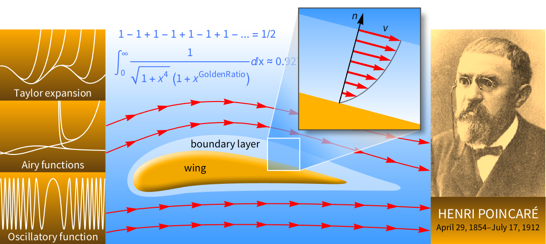Asymptotic expansions have played a key role in the development of fields such as aerodynamics, quantum physics and mathematical analysis, as they allow us to bridge the gap between intricate theories and practical calculations. Indeed, the leading term in such an expansion often gives more insight into the solution of a problem than a long and complicated exact solution. Version 11.3 of the Wolfram Language introduces two new functions, AsymptoticDSolveValue and AsymptoticIntegrate, which compute asymptotic expansions for differential equations and integrals, respectively. Here, I would like to give you an introduction to asymptotic expansions using these new functions.

The history of asymptotic expansions can be traced back to the seventeenth century, when Isaac Newton, Gottfried Leibniz and others used infinite series for computing derivatives and integrals in calculus. Infinite series continued to be used during the eighteenth century for computing tables of logarithms, power series representations of functions and the values of constants such as π. The mathematicians of this era were aware that many series that they encountered were divergent. However, they were dazzled by the power of divergent series for computing numerical approximations, as illustrated by the Stirling series for Gamma, and hence they adopted a pragmatic view on the issue of divergence. It was only in the nineteenth century that Augustin-Louis Cauchy and others gave a rigorous theory of convergence. Some of these rigorists regarded divergent series as the devil’s invention and sought to ban their use in mathematics forever! Fortunately, eighteenth-century pragmatism ultimately prevailed when Henri Poincaré introduced the notion of an asymptotic expansion in 1886.

Asymptotic expansions refer to formal series with the property that a truncation of such a series after a certain number of terms provides a good approximation for a function near a point. They include convergent power series as well as a wide variety of divergent series, some of which will appear in the discussion of AsymptoticDSolveValue and AsymptoticIntegrate that follows.

As a first example for AsymptoticDSolveValue, consider the linear differential equation for Cos: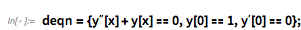✕ `deqn={(y^′′)[x]+y[x]==0,y==1,(y^′)==0};`

The following input returns a Taylor series expansion up to order 8 around 0 for the cosine function: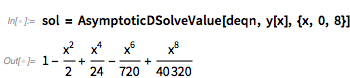✕ `sol = AsymptoticDSolveValue[deqn, y[x], {x, 0, 8}]`

Here is a plot that compares the approximate solution with the exact solution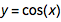: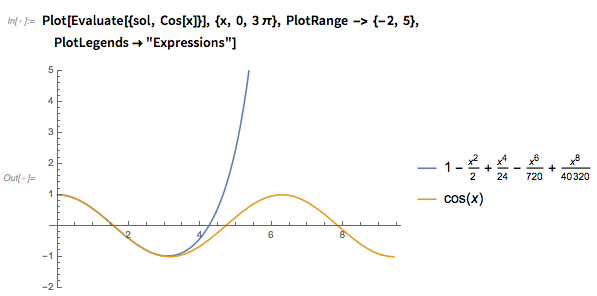✕ `Plot[Evaluate[{sol, Cos[x]}], {x, 0, 3 π}, PlotRange -> {-2, 5},PlotLegends->"Expressions"]`

Notice that the Taylor expansion agrees with the exact solution for a limited range of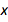near 0 (as required by the definition of an asymptotic expansion), but then starts to grow rapidly due to the polynomial nature of the approximation. In this case, one can get progressively better approximations simply by increasing the number of terms in the series. The approximate solution then wraps itself over larger portions of the graph for the exact solution: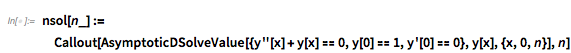✕ `nsol[n_]:=Callout[AsymptoticDSolveValue[{y''[x]+y[x]==0,y==1,y'==0},y[x],{x,0,n}],n]`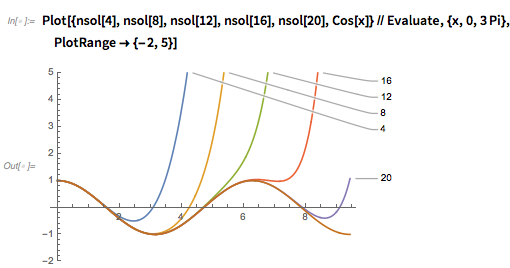✕ `Plot[{nsol,nsol,nsol,nsol,nsol,Cos[x]}//Evaluate,{x,0,3Pi},PlotRange->{-2,5}]`

Next, consider Bessel’s equation of order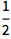, which is given by: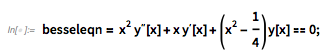✕ `besseleqn= x^2 (y^′′)[x]+x (y^′)[x]+(x^2-1/4) y[x]==0;`

This linear equation has a singularity at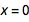in the sense that when, the order of the differential equation decreases because the term in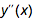becomes 0. However, this singularity is regarded as a mild problem because dividing each term in the equation by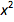results in a pole of order 1 in the term for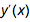and a pole of order 2 for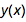. We say thatis a regular singular point for the differential equation and, in such cases, there is a Frobenius series solution that is computed here: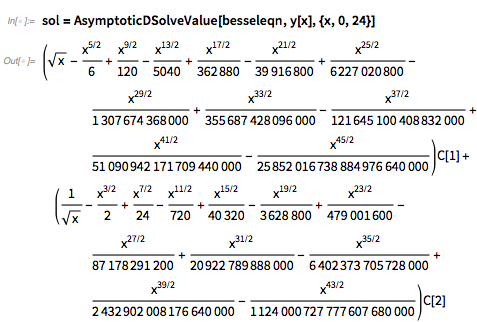✕ `sol=AsymptoticDSolveValue[besseleqn,y[x],{x,0,24}]`

Notice that there are fractional powers in the solution, and that only the second component has a singularity at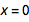. The following plot shows the regular and singular components of the solution: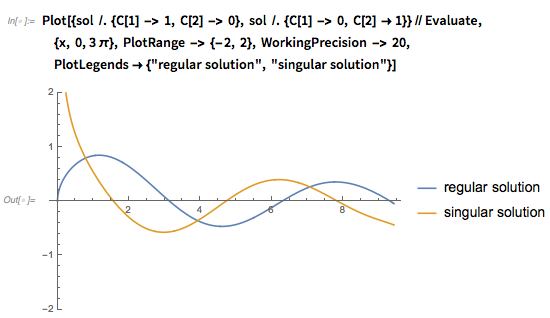✕ `Plot[{sol /. {C -> 1, C -> 0}, sol /. {C -> 0, C ->1}}//Evaluate, {x, 0,3π}, PlotRange -> {-2, 2}, WorkingPrecision -> 20,PlotLegends->{"regular solution", "singular solution"}]`

These solutions are implemented as BesselJ and BesselY, respectively, in the Wolfram Language, with a particular choice of constant multiplying factor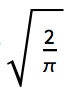: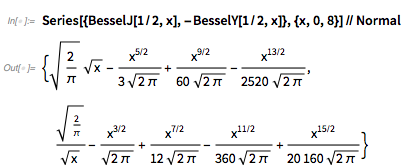✕ `Series[{BesselJ[1/2,x],-BesselY[1/2,x]},{x,0,8}]//Normal`

As a final example of a linear differential equation, let us consider the Airy equation, which is given by: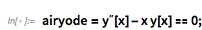✕ `airyode=(y^′′)[x]-x y[x]==0;`

This equation has an irregular singular point at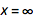, which may be seen by setting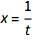, and then lettingapproach 0, so that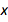approaches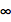. At such a point, one needs to go beyond the Frobenius scale, and the solution consists of asymptotic series with exponential factors: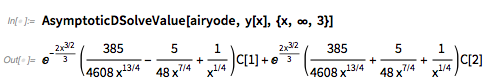✕ `AsymptoticDSolveValue[airyode, y[x], {x, ∞, 3}]`

The components of this solution correspond to the asymptotic expansions for AiryAi and AiryBi at Infinity: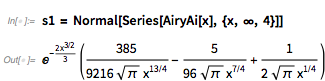✕ `s1 = Normal[Series[AiryAi[x], {x, ∞, 4}]]`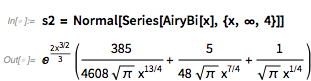✕ `s2 = Normal[Series[AiryBi[x], {x, ∞, 4}]]`

The following plot shows that the approximation is very good for large values of: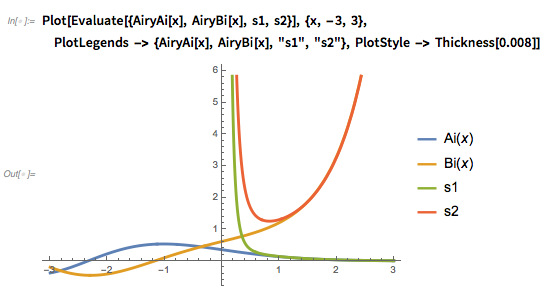✕ `Plot[Evaluate[{AiryAi[x], AiryBi[x], s1, s2}], {x, -3, 3}, PlotLegends -> {AiryAi[x], AiryBi[x], "s1", "s2"}, PlotStyle -> Thickness[0.008]]`

The asymptotic analysis of nonlinear differential equations is a very difficult problem in general. Perhaps the most useful result in this area is the Cauchy–Kovalevskaya theorem, which guarantees the existence of Taylor series solutions for initial value problems related to analytic differential equations. AsymptoticDSolveValue computes such a solution for the following first-order nonlinear differential with an initial condition. Quiet is used to suppress the message that there are really two branches of the solution in this case: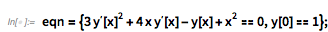✕ `eqn={3 (y^′)[x]^2+4 x (y^′)[x]-y[x]+x^2==0,y==1};`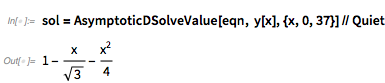✕ `sol=AsymptoticDSolveValue[eqn, y[x],{x,0,37}]//Quiet`

Notice that only three terms are returned in the solution shown, although 37 terms were requested in the input. This seems surprising at first, but the confusion is cleared when the solution is substituted in the equation, as in the following: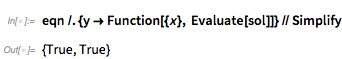✕ `eqn /. {y -> Function[{x}, Evaluate[sol]]} // Simplify`

Thus, the asymptotic expansion is actually an exact solution! This example shows that, occasionally, asymptotic methods can provide efficient means of finding solutions belonging to particular classes of functions. In that example, the asymptotic method gives an exact polynomial solution.

The examples that we have considered so far have involved expansions with respect to the independent variable. However, many problems in applied mathematics also involve a small or large parameter ϵ, and in this case, it is natural to consider asymptotic expansions with respect to the parameter. These problems are called perturbation problems and the parameter is called the perturbation parameter, since a change in its value may have a dramatic effect on the system.

Modern perturbation theory received a major impetus after the German engineer Ludwig Prandtl introduced the notion of a boundary layer for fluid flow around a surface to simplify the Navier–Stokes equations of fluid dynamics. Prandtl’s idea was to divide the flow field into two regions: one inside the boundary layer, dominated by viscosity and creating the majority of the drag; and one outside the boundary layer, where viscosity can be neglected without significant effects on the solution. The following animation shows the boundary layer in the case of smooth, laminar flow of a fluid around an aerofoil.

Prandtl’s work revolutionized the field of aerodynamics, and during the decades that followed, simple examples of perturbation problems were created to gain insight into the difficult mathematics underlying boundary layer theory. An important class of such examples are the so-called singular perturbation problems for ordinary differential equations, in which the order of the equation decreases when the perturbation parameter is set to 0. For instance, consider the following second-order boundary value problem: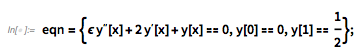✕ `eqn={ϵ (y^′′)[x]+2 (y^′)[x]+y[x]==0,y==0,y==1/2};`

When ϵ is 0, the order of the differential equation decreases from 2 to 1, and hence this is a singular perturbation problem. Next, for a fixed small value of the parameter, the nature of the solution depends on the relative scales forand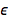, and the solution can be regarded as being composed of a boundary layer near the left endpoint 0, where ϵ is much larger than, and an outer region near the right endpoint 1, whereis much larger than. For this example, AsymptoticDSolveValue returns a perturbation solution with respect to: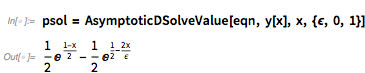✕ `psol = AsymptoticDSolveValue[eqn, y[x], x, {ϵ, 0, 1}]`

For this example, an exact solution can be computed using DSolveValue as follows: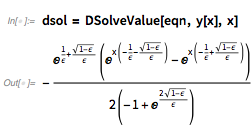✕ `dsol = DSolveValue[eqn, y[x], x]`

The exact solution is clearly more complicated than the leading term approximation from the perturbation expansion, and yet the two solutions agree in a very remarkable manner, as seen from the plots shown here (the exact solution has been shifted vertically by 0.011 to distinguish it from the approximation!):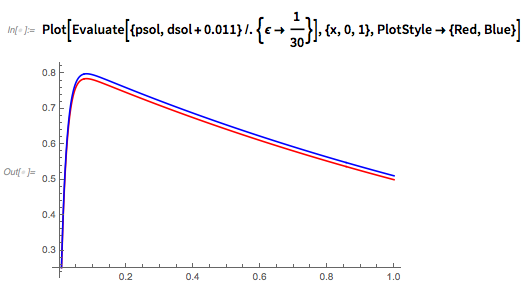✕ `Plot[Evaluate[{psol,dsol+0.011}/. {ϵ->1/30}],{x,0,1},PlotStyle->{Red,Blue}]`

In fact, the approximate solution approaches the exact solution asymptotically as ϵ approaches 0. More formally, these solutions are asymptotically equivalent: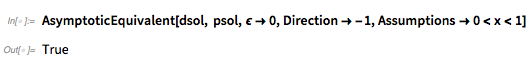✕ `AsymptoticEquivalent[dsol, psol,ϵ->0,Direction->-1,Assumptions->0

Asymptotic expansions also provide a powerful method for approximating integrals involving a parameter. For example, consider the following elliptic integral, which depends on the parameter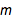: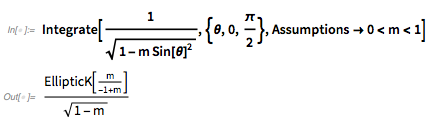✕ `Integrate[1/Sqrt[1-m Sin[θ]^2],{θ,0,π/2},Assumptions->0

The result is an analytic function offor small values of this parameter, and hence one can obtain the first five terms, say, of the Taylor series expansion using Series: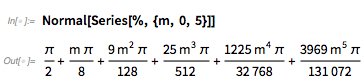✕ `Normal[Series[%, {m, 0, 5}]]`

The same result can be obtained using AsymptoticIntegrate by specifying the parameterin the third argument as follows: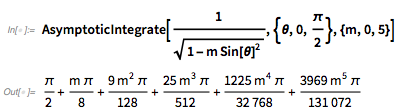✕ `AsymptoticIntegrate[1/Sqrt[1-m Sin[θ]^2],{θ,0,π/2},{m,0,5}]`

This technique of series expansions is quite robust and applies to a wide class of integrals. However, it does not exploit any specific properties of the integrand such as its maximum value, and hence the approximation may only be valid for a small range of parameter values.

In 1812, the French mathematician Pierre-Simon Laplace gave a powerful method for computing the leading term in the asymptotic expansion of an exponential integral depending on a parameter, whose integrand has a sharp peak on the interval of integration. Laplace argued that such an approximation could be obtained by performing a series expansion of the integrand around the maximum, where most of the area under the curve is likely to be concentrated. The following example illustrates Laplace’s method for an exponential function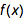with a sharp peak at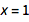: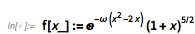✕ `f[x_]:=E^(-ω (x^2-2 x)) (1+x)^(5/2)`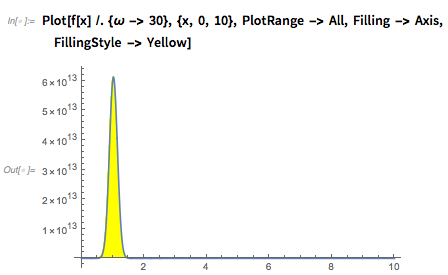✕ `Plot[f[x] /. {ω -> 30}, {x, 0, 10}, PlotRange -> All, Filling -> Axis, FillingStyle -> Yellow]`

Laplace’s method gives the following simple result for the leading term in the integral of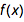from 0 to Infinity, for large values of the parameter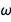: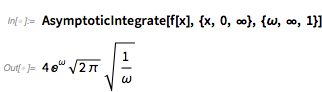✕ `AsymptoticIntegrate[f[x], {x, 0, ∞}, {ω, ∞, 1}]`

The following inputs compare the value of the approximation for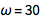with the numerical result given by NIntegrate: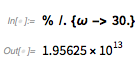✕ `% /. {ω -> 30.}`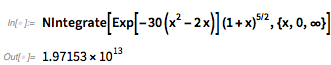✕ `NIntegrate[Exp[-30 (x^2-2 x)] (1+x)^(5/2),{x,0,∞}]`

The leading term approximation is reasonably accurate, but one can obtain a better approximation by computing an extra term: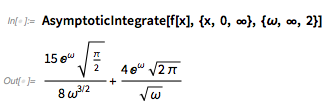✕ `AsymptoticIntegrate[f[x], {x, 0, ∞}, {ω, ∞, 2}]`

The approximate answer now agrees very closely with the result from NIntegrate: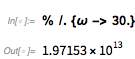✕ `% /. {ω -> 30.}`

The British mathematicians Sir George Gabriel Stokes and Lord Kelvin modified Laplace’s method so that it applies to oscillatory integrals in which the phase (exponent of the oscillatory factor) depends on a parameter. The essential idea of their method is to exploit the cancellation of sinusoids for large values of the parameter everywhere except in a neighborhood of stationary points for the phase. Hence this technique is called the method of stationary phase. As an illustration of this approach, consider the oscillatory function defined by: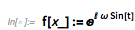✕ `f[x_]:=E^(I ω Sin[t])`

The following plot of the real part of this function for a large value ofshows the cancellations except in the neighborhood of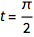, where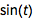has a maximum: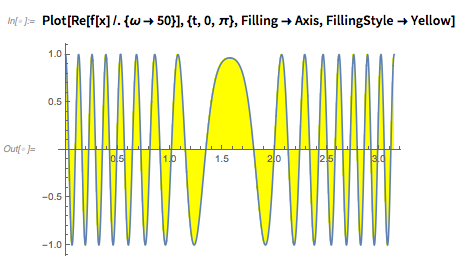✕ `Plot[Re[f[x]/. {ω->50}],{t,0,π},Filling->Axis,FillingStyle->Yellow]`

The method of stationary phase gives a first-order approximation for this integral: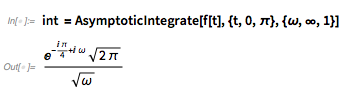✕ `int =AsymptoticIntegrate[f[t],{t,0,π},{ω,∞,1}]`

This rather simple approximation compares quite well with the result from numerical integration for a large value of: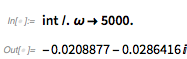✕ `int/. ω->5000.`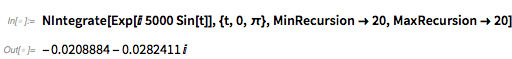✕ `NIntegrate[Exp[I 5000 Sin[t]],{t,0,π},MinRecursion->20,MaxRecursion->20]`

As noted in the introduction, a divergent asymptotic expansion can still provide a useful approximation for a problem. We will illustrate this idea by using the following example, which computes eight terms in the expansion for an integral with respect to the parameter: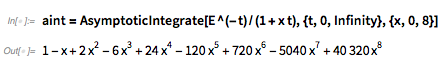✕ `aint=AsymptoticIntegrate[E^(-t)/(1+x t),{t,0,Infinity},{x,0,8}]`

The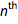term in the asymptotic expansion is given by: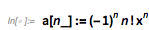✕ `a[n_]:=(-1)^n n! x^n`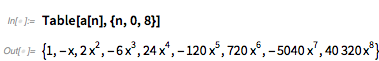✕ `Table[a[n],{n,0,8}]`

SumConvergence informs us that this series is divergent for all nonzero values of: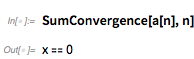✕ `SumConvergence[a[n],n]`

However, for any fixed value ofsufficiently near 0 (say,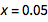), the truncated series gives a very good approximation:✕ `aint/.x-> 0.05`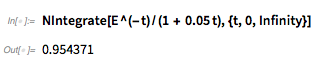✕ `NIntegrate[E^(-t)/(1 + 0.05 t),{t,0,Infinity}]`

On the other hand, the approximation gives very poor results for the same value ofwhen we take a large number of terms, as in the case of 150 terms: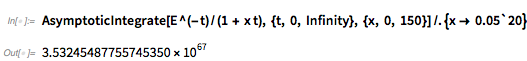✕ `AsymptoticIntegrate[E^(-t)/(1 + x t), {t, 0, Infinity}, {x, 0, 150}]/.{x-> 0.05`20}`

Thus, a divergent asymptotic expansion will provide excellent approximations if we make a judicious choice for the number of terms. Contrary to the case of convergent series, the approximation typically does not improve with the number of terms, i.e. more is not always better!

Finally, we note that the exact result for this integral can be obtained either by using Integrate or Borel regularization: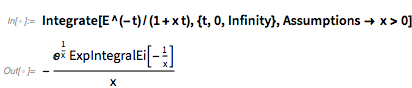✕ `Integrate[E^(-t)/(1+x t),{t,0,Infinity},Assumptions-> x>0]`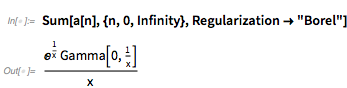✕ `Sum[a[n],{n,0,Infinity},Regularization->"Borel"]`

Both these results give essentially the same numerical value as the asymptotic expansion with eight terms: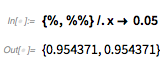✕ `{%,%%}/.x-> 0.05`

In connection with the previous example, it is worth mentioning that Dutch mathematician Thomas Jan Stieltjes studied divergent series related to various integrals in his PhD thesis from 1886, and is regarded as one of the founders of asymptotic expansions along with Henri Poincaré.

As a concluding example for asymptotic approximations of integrals, consider the following definite integral involving GoldenRatio, which cannot be done in the sense that an answer cannot presently be found using Integrate: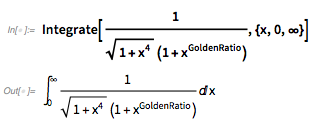✕ `Integrate[1/(Sqrt[1+x^4](1+x^GoldenRatio)),{x,0,∞}]`

This example was sent to me by an advanced user, John Snyder, shortly after the release of Version 11.3. John, who is always interested in trying new features after each release, decided to try the example using AsymptoticIntegrate after replacing GoldenRatio with a parameter α, as shown here: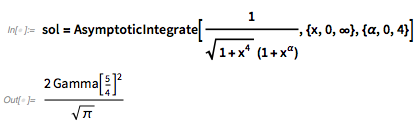✕ `sol=AsymptoticIntegrate[1/(Sqrt[1+x^4](1+x^α)),{x,0,∞},{α,0,4}]`

He noticed that the result is independent of α, and soon realized that the GoldenRatio in the original integrand is just a red herring. He confirmed this by verifying that the value of the approximation up to 80 decimal places agrees with the result from numerical integration: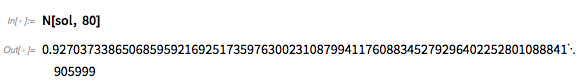✕ `N[sol, 80]`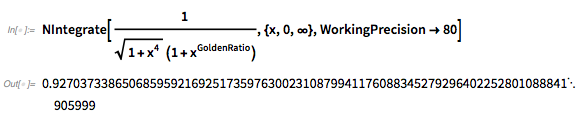✕ `NIntegrate[1/(Sqrt[1+x^4](1+x^GoldenRatio)),{x,0,∞},WorkingPrecision->80]`

Finally, as noted by John, the published solution for the integral is exactly equal to the asymptotic result. So AsymptoticIntegrate has allowed us to compute an exact solution with essentially no effort!

Surprising results such as this one suggest that asymptotic expansions are an excellent tool for experimentation and discovery using the Wolfram Language, and we at Wolfram look forward to developing functions for asymptotic expansions of sums, difference equations and algebraic equations in Version 12.

I hope that you have enjoyed this brief introduction to asymptotic expansions and encourage you to download a trial version of Version 11.3 to try out the examples in the post. An upcoming post will discuss asymptotic relations, which are used extensively in computer science and elsewhere.